# Time Series Data Chapter Exam

Exam Instructions:

Choose your answers to the questions and click 'Next' to see the next set of questions. You can skip questions if you would like and come back to them later with the yellow "Go To First Skipped Question" button. When you have completed the practice exam, a green submit button will appear. Click it to see your results. Good luck!

### Page 1

#### Question 1 1. What is the general trend in global ocean heat content?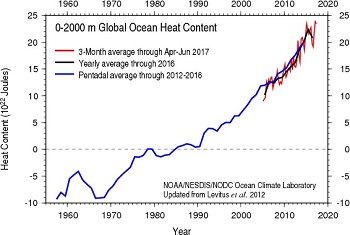#### Question 5 5. What is significant about 2007 in the time series plot for the value of OMX Iceland?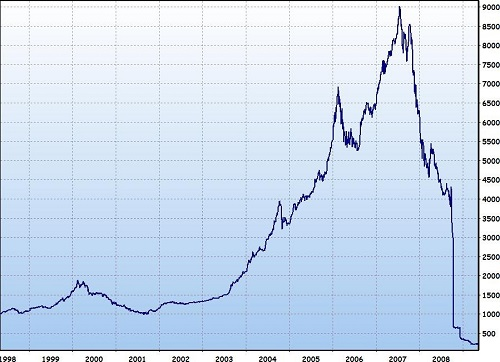### Page 2

#### Question 6 6. Given the following table of data of sales vs. time, what might the graph of the time plot of sales vs time with its 5-mean smoothed time plot superimposed look like?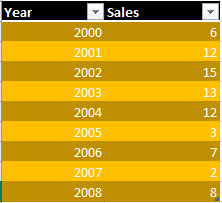#### Question 7 7. Given the following time plot of sales vs. time, do you suppose that the 3-mean smoothed plot will show an increasing or decreasing trend with regard to sales vs. time?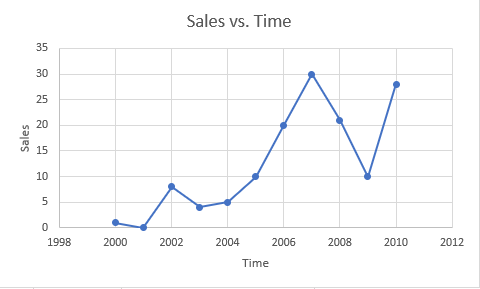#### Question 8 8. Given the following table of data of sales vs. time, what might the graph of the time plot of sales vs time with its 3-mean smoothed time plot superimposed look like?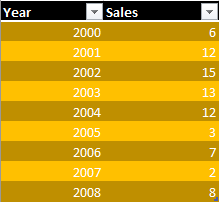#### Question 9 9. Given the following data, what would the general shape of the time plot of sales vs. years, with a 3 and 5-mean smoothed plot superimposed, most look like, given the following choices? (The original data is in blue.)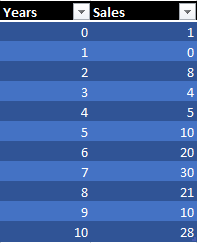#### Question 10 10. Given the following time plot of sales vs. time, do you suppose that the 5-mean smoothed plot will show an increasing or decreasing trend with regard to sales vs. time?### Page 3

#### Question 11 11. What is the projected value for the second quarter in year 3? (Deseasonalize the given data, then create a regression line to fit.)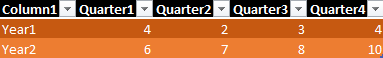#### Question 12 12. Given the following table of seasonal (or quarterly) numbers over year 1 and year 2, what is the seasonal indice for quarter 1?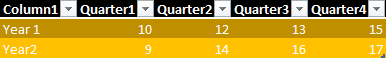#### Question 13 13. Given the following table of seasonal (or quarterly) numbers over year 1 and year 2, what is the deseasonalized number for quarter 1?#### Question 14 14. Given the following data set for a time plot, what would be a linear regression (or least squares regression) that best represents the deseasonalized trend line?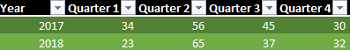#### Question 15 15. Given the following sales data through the eight quarters of 2017 and 2018, what would the time plot of this sales data look like with a deseasonalized time plot superimposed?### Page 4

#### Question 16 16. Given the following data -with dollar amounts rounded to the tenths place, what would be the mean of the first 3 dollar amounts?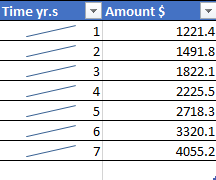#### Question 17 17. Given the following data -with dollar amounts rounded to the tenths place, what would be the mean of the first 5 dollar amounts?#### Question 18 18. What might the following time plot of sales vs. years look like with its 3-mean smoothed plot superimposed?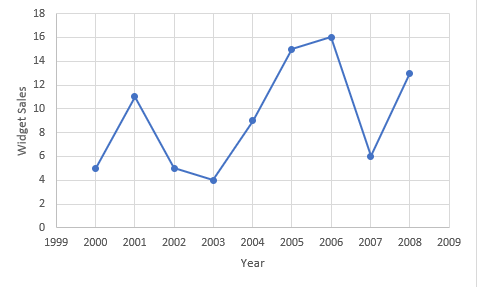#### Question 19 19. What would the sales data below look like if it were numerically smooth it using 3-mean smoothing?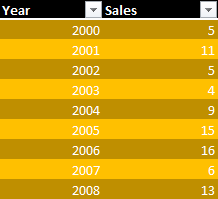#### Question 20 20. What might the following time plot of sales vs. years look like with its 5-mean smoothed plot superimposed?#### Time Series Data Chapter Exam Instructions

Choose your answers to the questions and click 'Next' to see the next set of questions. You can skip questions if you would like and come back to them later with the yellow "Go To First Skipped Question" button. When you have completed the practice exam, a green submit button will appear. Click it to see your results. Good luck!

Support